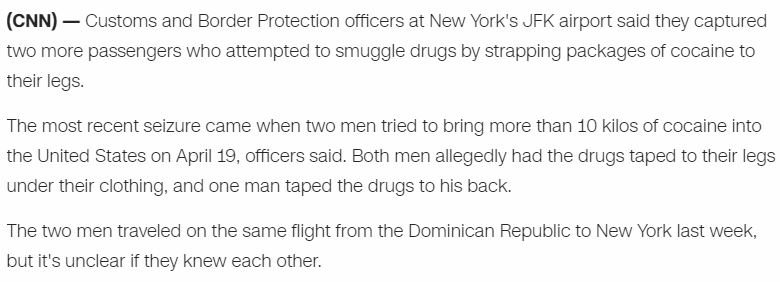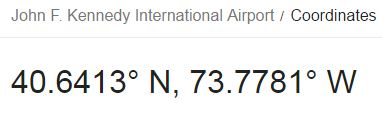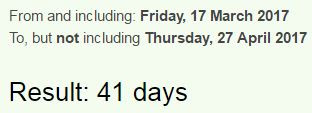CipherApr 27, 2017

CNN - Airline passengers caught with 10 kilos of cocaine in pants... absolute joke

Today is April 27th and here is another hilarious story that took place on April 19th... I don't know how they can get away with these stories but anyone that ever got on a plane would realize that you would never get away with taping cocaine to your legs...

ten kilograms = 20+5+14 + 11+9+12+15+7+18+1+13+19 = 144 (English Ordinal)
Note at what Time the story was updatedAs always they give us something nice in the title (7x7x7 = 343)April 19th was a date with 33 numerology (4+19+ 2+0+1+7 = 33)
They tried to smuggle 10 kilos of cocaine
smuggle = 8+5+6+2+2+6+4 = 33 (Reverse Reduction)
ten kilos = 2+5+5 + 2+9+3+6+1 = 33 (Full Reduction)
In the statement, it was not mentioned what tipped off the officers to conduct additional inspections on the men. But they were taken to a private search room where officers seized 23 pounds (10.4 kilos) of cocaine estimated at a nearly \$400,000 street value.
twenty three pounds = 2+5+5+5+2+7 + 2+8+9+5+5 + 7+6+3+5+4+10 = 90 (Single Reduction)
ninety = 5+9+5+5+2+7 = 33 (Full Reduction)
Also they are saying that it's nearly worth 400,000 dollars in street value33rd prime number is 137

They took 23 pounds on 4/19 = 4+19 = 23, the story is coming out on CNN today on a date with 23 numerology (4+2+7+2+0+1+7 = 23)
cocaine = 3+6+3+1+9+5+5 = 32 (Full Reduction)

cocaine = 6+3+6+8+9+4+4 = 40 (Reverse Reduction), they were caught on JFK airport located at 40°North latitudeLast month at the same airport, officers said they discovered two other passengers on separate days with drugs taped under their pants.The last time they reported something like this was on March 17th and this stupid story is released on the 41st day from March 17th on a date with 41 numerology (4+27 + 2+0+1+7  = 41)If we don't count the end date it's a 40 day span connecting to cocaine = 6+3+6+8+9+4+4 = 40 (Reverse Reduction)

The guys that were caught on the 19th traveled on the same flight from "Dominican Republic to New York" = 4+15+13+9+14+9+3+1+14 + 18+5+16+21+2+12+9+3 + 20+15 + 14+5+23 + 25+15+18+11 = 314 (English Ordinal)